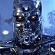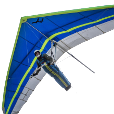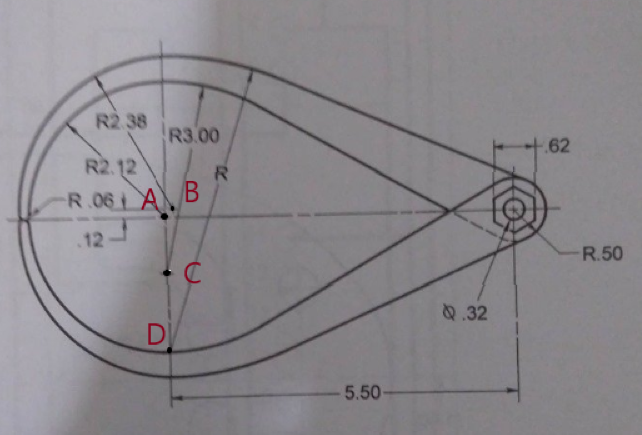# Need help in drawing exercise## Recommended Posts##### Share on other sitesYou will need to draw several circles. Connect the circles with lines using the Tangent-Tangent snap. Use the lines to trim the circles. Some of the dimensions you will need are missing, but you can calculate them using the numbers you do have.

##### Share on other sitesPost a copy of what you have drawn so far.  I'm not sure you can calculate all the missing numbers needed to fully construct the drawing in question.  But that's just my opinion.  I could be wrong.

##### Share on other sitesThe inner upper edge can be easily created with circles of R2.12,  R3, and R.5. then adding a line tangent to the R3 and R.5 circles.

The outer profile may need the assumption that R is center at a point 2.12 below A. Draw a circle of R2.38 centered at B.  Now draw a line through D and B. The intersection of this line with the R2.38 circle can be used to determine the value of R.   Draw a circle of radius R centered at D then a line tangent to it and the R.5 circle.##### Share on other sites12 hours ago, lrm said:

........... Draw a circle of R2.38 centered at B.

How would you arrive at B?

##### Share on other sites3 hours ago, eldon said:

How would you arrive at B?

Good question! Fudge an x coordinate, perhaps 0.12 from A.  Not the best drawing exercise.

##### Share on other sites0.12 the height above A is given and the same to the right (R0.06 at the outer edge is given)

##### Share on other sitesI think that it can be derived from drawing, and I reckon it is 0.14004985 to the right.

I would draw the figure by starting with the 2.12r circle.

Then by offsetting this circle and the centre line by 0.06 each to get the centre of the 0.06 circle.

Then draw the 0.06r circle and offset this circle by 2.26, and where this intersects with an offset of 0.12 from the centre line, is point B.

##### Share on other sitesIt works best for me if I assume the dimensions shown have been rounded, so instead of .12 I use .125, instead of .06 I use .0625, instead of 2.38 I use 2.375.

##### Share on other sitesThat would be the easiest way to go.

Unfortunately, with these exercises, there is a note:- 'If dimensions are given, create your drawing geometry exactly to the specified dimensions.'

##### Share on other sitesOn ‎8‎/‎17‎/‎2019 at 1:29 AM, eldon said:

How would you arrive at B?

I derived B with parametrics.  After drawing the arc of 2.12 I drew the .06 circle close by where it will go, set some parameters so the existing arc and line wouldn't move, set the .06 circle tangent to the arc and horizontal line.  Then I drew a 2.38 circle close to where it goes, set the left side of the circle tangent to the left side of the .06 circle, then set a coincident paramenter for the center of the 2.38 circle and the horizontal line set above the center line by the given amount of .12.  The distance to the horizontal center line is .14002743.

##### Share on other sitesThat seems to be near enough!

##### Share on other sitesIf you assume that the R0.06 radius is tangent to the horizontal centerline then you can use circle TTR to position it as shown below. (tangent to the line and the R2.12 arc).  We know the y coordinate of B is 0.12.  The x coordinate can be found by drawing a concentric circle of R 2.32 ( = 2.38 - 0.06) to the 0.06 circle. The intersection of this circle and the 0.12 offset line is the location of B.

##### Share on other sitesYeah when you draw it out then 0.14 makes good sense. And it doesn't need any other dimensions than those given.

##### Share on other sites55 minutes ago, lrm said:

If you assume that the R0.06 radius is tangent to the horizontal centerline then you can use circle TTR to position it as shown below. (tangent to the line and the R2.12 arc).  We know the y coordinate of B is 0.12.  The x coordinate can be found by drawing a concentric circle of R 2.32 ( = 2.38 - 0.06) to the 0.06 circle. The intersection of this circle and the 0.12 offset line is the location of B.

Doing that way is a very close approximation and probably close enough.  Because of the 2.38 arc, etc. the tangent  point on the .06 radius is not at the quadrant but actually lower, and my method will get you to the actual tangent point.

##### Share on other sites2 hours ago, rkent said:

Doing that way is a very close approximation and probably close enough.  Because of the 2.38 arc, etc. the tangent  point on the .06 radius is not at the quadrant but actually lower, and my method will get you to the actual tangent point.

I respectfully disagree. It is an exact solution.

Centering a circle of R2.38 (blue) anywhere on the circumference of the R2.32 circle (yellow) will position the circle tangent to the R0.06 circle. I agree that since B is above the center of the R0.06 circle the tangent point will be below its center (consistent with my post).  Why did you mention quadrant?

•1
##### Share on other sitesAs long as the OP has enough information to enable the exercise to be drawn.

## Join the conversation

You can post now and register later. If you have an account, sign in now to post with your account.
Note: Your post will require moderator approval before it will be visible.×   Pasted as rich text.   Restore formatting

Only 75 emoji are allowed.